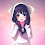## Monday 7 January 2019

Return on Investment that is often abbreviated with the ROI is the ratio of profitability that measures the efficiency of an investment by comparing with the total net profit costs or capital invested. In other words, return on Investment or ROI measures the profit or loss generated from investments against the amount of money invested.

The return on Investment (ROI) is often referred to as return on investment or the return on investment.

Return on Investment or ROI is one of the most common approach used to evaluate the financial consequences of a business investment decisions and actions. ROI can be used for personal financial decisions, comparing the company's profitability or to compare efficiency investments. If the results of the calculation of the Return on Investment or ROI at an investment plan is positive and there are no more opportunities to obtain higher ROI results again so that investment can be made.

### Basically, return on Investment or ROI it is important for the financial ratios:

1. Make the purchasing decisions of assets (buildings, computers, vehicles, and machinery production)

2. Funding for project decision making and different types of program (e.g. program recruitment, training and marketing programs)

3. Make stock investment decisions or investments in venture capital (venture capital)

### How to calculate Return on Investment (ROI) return on investment

Here is how to calculate Return on Investment (ROI) with the formula and example case.

### Formula ROI (Return on Investment)

Return on Investment (ROI) return on investment is calculated by means of reducing the cost of investment of Total income and divide it by the total cost of the investment. The result of the calculation of the ROI of this can be the percentage or ratio. The following is the equation or formula Return on Investment (ROI) return on investment:

ROI = (income from Investments – Investment Cost)/cost of investment

Please note that the define and the return on investment formula or a return on investment or ROI can be modified to suit the circumstances and are basically depends on what is being entered as a refund (return) and costs (cost). For example, a production manager will probably use these ROI formulas for calculating the rate of return a production machine that will be bought while an investor will probably use this ROI formula to calculate return on Its Stock Investments. So in other words, the formula ROI can be very flexible depending on what is measured or what is shown to the user.

### An example of the calculation of the Return on Investment (ROI) return on investment

The following are two examples of the calculation of the Return on Investment or ROI.

#### Example 1

An Investor bought the stock as much as 50,000 shares at a price of \$ 1,000 per shares. A year later, the Investor sells the shares valued at \$ 2,500 per shares. What is the ROI on these stocks?

Note:

Income from Investments = \$125 million,-(from the calculation of the 50,000 x \$2,500,-)
Investment Costs = \$50 million,-(from the calculation of the 50,000 x \$1,000,-)
ROI =?

Solution:

ROI = (125 million – 50 million)/50 million
= 75 million/50 million ROI
ROI = 1.5 or 150%

Return on Investment or ROI on shares bought the Investor is 1.5 times or 150%.

#### Example 2

A production manager wanted to buy packing machine for \$160 million. With the packing machine, production manpower deployment can save you as much as 9 people. The salaries of every labor is \$ 3 million. What is the ROI for the packing machine for a year?

Note:

Income from Investments = \$ 324 million,-(from 3 million x 9 x 12 months)
= \$ Investment Cost 160 million,-
= ROI?

(1) ROI = (324 million – 160 million)/160 million
(2) ROI = (164 million)/160 million
(3) ROI = 1.025 or 102.5%

So the return on investment or ROI Machine Packing is of 1.025 times or 102.5%.

### Analysis and assessment of the Return on Investment (return on investment) or ROI

Generally, any investment which is worth a positive ROI can be consider as an investment that gives a good return. Positive ROI indicates that total investment costs can be restore and also be able to earn profit from the rest of the cost of the investment.

While a negative ROI indicates revenue that gets cannot cover the total cost of the investment grant. Thus, it can be said that the rate of return or higher ROI will be better than the rate of return or the low ROI.

Calculation of the ROI is very flexible and can be use for any investment. Management companies can use this to measure ROI return on invest capital, investors can use it to measure the performance of stocks they invested while Individuals can use this Return on Investment for measure the return on their assets.

#### 1 comment:

1.